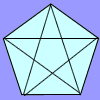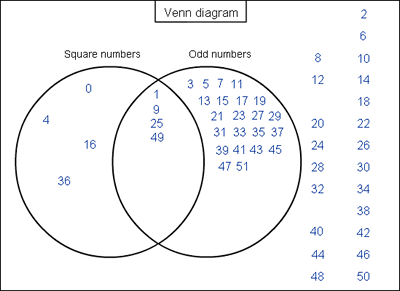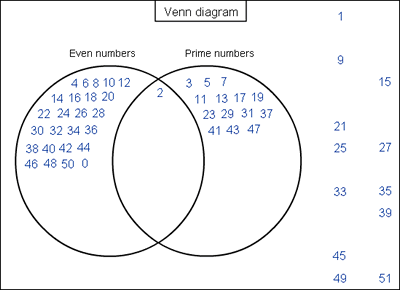#### You may also like### Number Detective

Follow the clues to find the mystery number.### Prime Magic

Place the numbers 1, 2, 3,..., 9 one on each square of a 3 by 3 grid so that all the rows and columns add up to a prime number. How many different solutions can you find?### Diagonal Trace

You can trace over all of the diagonals of a pentagon without lifting your pencil and without going over any more than once. Can the same thing be done with a hexagon or with a heptagon?

# Various Venns

##### Age 7 to 11 Challenge Level:

Cong from St Peter's Roman Catholic Primary School sent in solutions to both parts of this problem. Cong sent us pictures of his completed Venn diagrams. Here is the first:And here is the second:I am interested in where you have placed zero in both Venn diagrams, Cong. It is difficult to say whether 0 is odd or even - I can see why you have placed it as an even number, though, as it seems to extend the pattern $8, 6, 4, 2, ... 0$. It's easy to forget that $0$ is a square number - but certainly $0 \times 0=0$.

Michael from Kings Elementary School explained a bit how he went about filling in the first Venn diagram. Michael assumes that 0 is not a square number:

In order to find the solution to this problem you first need to list the squares which are $49, 36, 25, 16, 9, 4$ and $1$. There are $4$ odd square numbers which are $49, 25, 9$, and $1$. So you put the rest of the squares in the square numbers and odd numbers in the odd section and anything else you don't do anything with it.

Well done! Eliza, from Danebank School, also left $0$ out of the Venn diagram. What do you think? Is $0$ square and even?